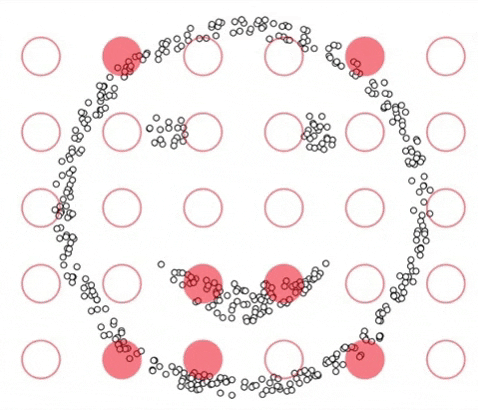Clustering is an unsupervised learning technique that finds patterns in data without being explicitly told what pattern to find.DBSCAN does this by measuring the distance each point is from one another, and if enough points are close enough together, then DBSCAN will classify it as a new cluster.As seen above, there are two distinct clusters in the Test Data. KMeans, another popular clustering technique, fails to accurately cluster this data because KMeans creates a linearly separable boundary between clusters when k=2.

DBSCAN instead defines clusters based on two parameters: Epsilon and Min_Points

• Epsilon — The maximum distance a point can be from another point to be considered a neighbor.

• Min_Points — The amount of points needed within the range of epsilon to be considered a cluster.

### Benefits of DBSCAN

#### It requires minimal domain knowledge to determine the input parameters.

Other clustering algorithms like KMeans requires the user to know how many clusters exist in the data.

Instead of requiring how many clusters should be found, DBSCAN requires the user to input the maximum distance apart each point of data can be to be considered part of a cluster and how many data points it takes to form a cluster.

#### It discovers clusters of any shape.

Since DBSCAN creates clusters based on epsilon and the number of neighbors each point has, it can find clusters of any shape. DBSCAN works best when the clusters are of the same density (distance between points). When clusters of varying density are present, this can make it hard for DBSCAN to identify the clusters.

``````# Download the test package
pip install -i https://test.pypi.org/simple/ dbscan2==0.0.3

# Import it!
from dbscan2 import dbscan2

# If you would like to plot the results import the following
from sklearn.datasets import make_moons
import pandas as pd
``````

To understand and implement DBSCAN from scratch, we will need to know how DBSCAN is clustering the data. Along with Epsilon and Min Points, there are three more essential terms to understand:

• Noise — This is a point that does not have enough neighbors within epsilon to be part of a cluster (including itself).

• Border Points — This is a point that has neighbors within epsilon but not enough neighbors to be a core point. These points make up the edge of the cluster.

• Core Points — Points that have the Min Points required within epsilon (including itself). These points along with border points will form a cluster.We are going to implement DBSCAN using a Class and call it dbscan2. It will have two main methods: fit and predict.

``````def __init__()
``````

The class will be initialized with standardized two feature array, epsilon, and the number of points required to create a cluster. It will also be initialized with a cluster label and a noise label.

``````class dbscan2():
def __init__(self,df, epsilon=1, min_points=5):
self.df = np.array(df)
self.epsilon = epsilon
self.min_points = min_points
self.cluster_label = 0
self.noise = 0
``````

#### Helper Functions

We will use euclidean distance to measure how far each point is from one another. Euclidean distance will measure the ordinary straight line distance from one pair of coordinates to another pair.``````def dist(self, point1, point2):
"""Euclid distance function"""
x1 = point1
x2 = point2
y1 = point1
y2 = point2
# create the points
p1 = (x1 - x2)**2
p2 = (y1 - y2)**2
return np.sqrt(p1 + p2)
``````

The other helper function we will need will be called rangeQuery. The function will help us find out how many neighbors each point has that is within epsilon.

``````def rangeQuery(self, x):
"""Query database against x and return all points that are <=
epsilon"""
neighbors = []
for y in range(len(self.df)):
q = self.df[y, :2]
# If the distance is <= than epsilon then append to neighbors list
if self.dist(x, q) <= self.epsilon:
neighbors.append(y)
return neighbors
``````
``````def fit()
``````

Our fit function will iterate through the entire dataset and determine how many neighbors each point in our dataset has.

If a single point does not have enough neighbors (neighbors < min_points), then it will be labeled as noise.

If a single point has enough neighbors (neighbors ≥ min_points) then the point will be assigned a new cluster label, and all of its neighbors will also be given the same label. The fit function will enter a while loops adding all the neighbors to a Queue so that they can be correctly labeled as part of the new cluster along with the neighbors of newly found neighbors.

This process will continue until the algorithm has examined all points.

``````def predict():
``````

When making a prediction, the algorithm will identify if the new input point has any neighbors using the rangeQuery. If it does, then the new point will be predicted to have the same label as its neighbors.

#### How does it compare to the Scikit-Learn version?As expected, our implementation from scratch ends up with the same results as the Scikit-Learn version. Our version of DBSCAN does take longer, and I would still use Scikit-Learns version, but hopefully implementing the algorithm from scratch helped you better understand how arbitrary cluster shapes are found using DBSCAN.

Find the code for the blog here

A Density-Based Algorithm for Discovering Clusters in Large Spatial Databases with Noise

DBSCAN

Euclidean Distance